# Vapour Pressure of a Liquid Solution:

The liquid solution is formed when a solvent is in a liquid phase. The solute may be solid, liquid or gas. On the basis of solute, the liquid solution is classified into 3 types as:

• Solid in liquid
• Liquid in liquid
• Gas in liquid

We will discuss the various properties of liquid in liquid solution and solid in liquid solutions.

## Vapour Pressure of a Liquid-Liquid Solution:

Let us take a binary solution made up of two volatile liquids 1 and 2. As the liquid start evaporating; a stage will come when the vapour pressure of liquid will be in equilibrium with the corresponding liquid. As we know that vapour pressure of liquid is proportional to its mole fraction.  The quantitative relationship between the vapour pressure and mole fraction in binary solution is given by Roult’s Law.

### Roult’s Law:

It states that for the solutions of volatile liquid, the partial vapour pressure of each component of the solution is directly proportional to its mole fraction present in solution.According to Dalton’s Law of partial pressure, The total pressure (ptotal) over the solution phase in the container will be the sum of partial pressure of the components in solution. If the solution is made up of two volatile liquids then total pressure above the solution is: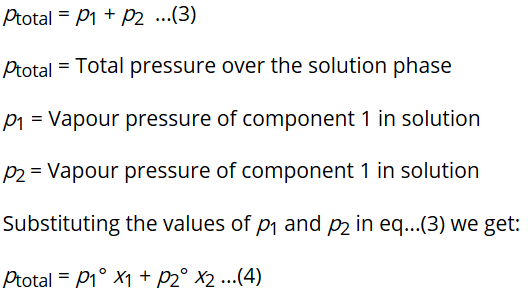As total mole fraction of components in any solution is 1. Therefore,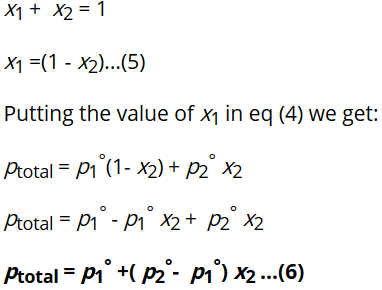The equation (6) is known as the mathematical expression of Roult’s Law for the solution made up of two volatile liquids.

From this equation (6) we can conclude the following points :

• ptotal can be related to mole fraction of any of the one component.
• ptotal varies linearly with x1.
• Ptotal increases or decreases with increase of x1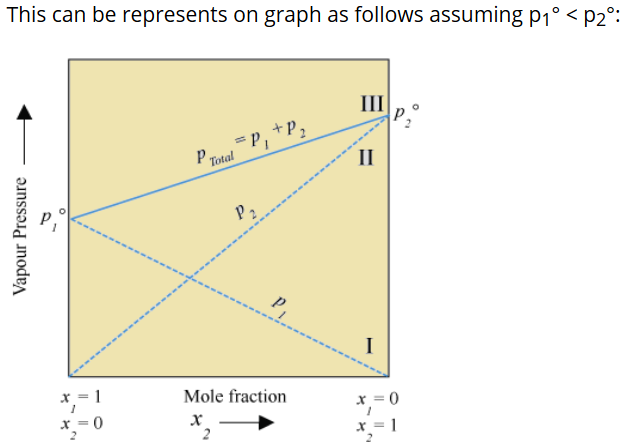### The conclusion from the graph: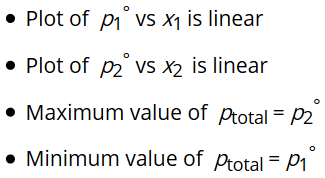The composition of vapour phase at equilibrium is determined by using Dalton’s Law. Let y1 and y2 be the mole fraction of liquid 1 and 2 in solution then partial vapour pressure of each component is written as: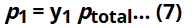Equation (7) gives you the value of partial vapour pressure of each component in a vapour phase.